Study Materials

# NCERT Solutions for Class 9th Mathematics

Page 2 of 2

## Chapter 12. Herons Formula

### Exercise 12.2

Q1: A park, in the shape of a quadrilateral ABCD, has ∠ C = 90º, AB = 9 m, BC = 12 m, CD = 5 m and AD = 8 m. How much area does it occupy?

Solution: Let us join BD.In ΔBCD, applying Pythagoras theorem,

BD2 = BC2 + CD2

= (12)2 + (5)2

= 144 + 25

BD2 = 169

BD = 13 m

Area of ΔBCD

For ΔABD,

By Heron's formula,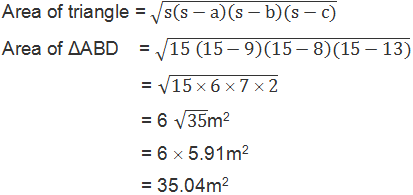Area of Quadrilateral = Area of ΔABD + Area of ΔBCD

= 30m2+ 35.04m2

= 65.04m2

Q2: Find the area of a quadrilateral ABCD in which AB = 3 cm, BC = 4 cm, CD = 4 cm, DA = 5 cm and AC = 5 cm.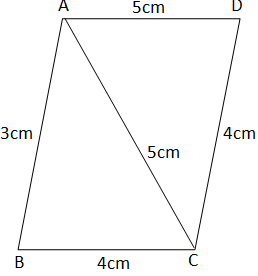Solution:

In ΔABC

AB = 3cm, BC = 4cm, AC = 5cm

Area of ΔABC

For ΔADC,

Perimeter = 2s = AC + CD + DA = (5 + 4 + 5) cm = 14 cm

s = 7 cm

By Heron's formula,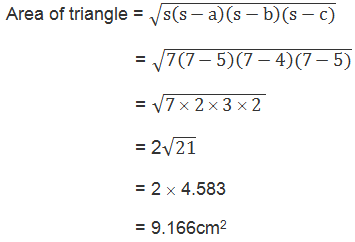Area of ABCD  = Area of ΔABC + Area of ΔADC

= 6 + 9.166

= 15.166 = 15.2cm2

Q3: Radha made a picture of an aero plane with colored papers as shown in the given figure. Find the total area of the paper used.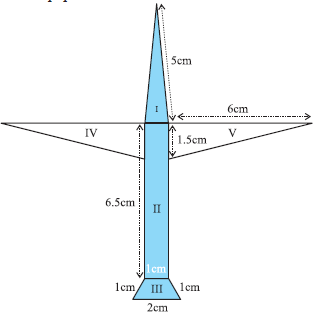Solution:

For triangle I

This triangle is an isosceles triangle.

Perimeter = 2s = (5 + 5 + 1) cm = 11cm

S =  = 5.5cm

Area of the triangle

For quadrilateral II

This quadrilateral is a rectangle.

Area = l × b = (6.5 × 1) cm2 = 6.5 cm2

For quadrilateral III

This quadrilateral is a trapezium.

Perpendicular height of parallelogram

Area = Area of parallelogram + Area of equilateral triangle

= 0.866 + 0.433 = 1.299 cm2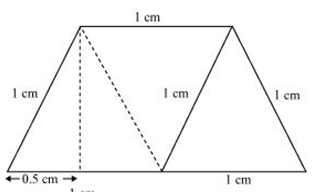Area of triangle (IV) = Area of triangle in (V)

Total area of the paper used = 2.488 + 6.5 + 1.299 + 4.5 × 2

= 19.287 cm2

Q4: A triangle and a parallelogram have the same base and the same area. If the sides of the triangle are 26 cm, 28 cm and 30 cm, and the  parallelogram stands on the base 28 cm, find the height of the parallelogram.

Solution:

Here, Sides of triangle be A, B, C.

A = 26cm, b = 28cm, c = 30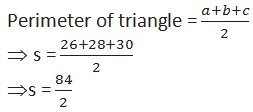⇒ s = 42cm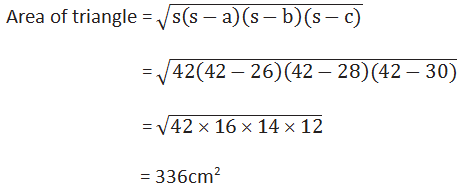Area of parallelogram = Area of triangle

28cm × h = 336 cm2

h = 336/28 cm

h = 12 cm

The height of the parallelogram is 12 cm.

Q5: A rhombus shaped field has green grass for 18 cows to graze. If each
side of the rhombus is 30 m and its longer diagonal is 48 m, how much
area of grass field will each cow be getting?

Solution:

Diagonal AC divides the rhombus ABCD into two congruent triangles of
equal area.

Semi perimeter of ΔABC = (30 + 30 + 48)/2 m = 54 m

Using heron's formula,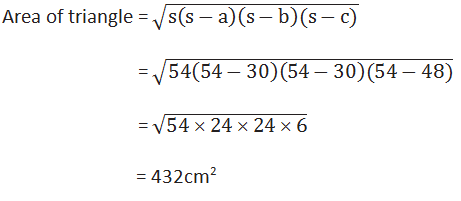Area of field = 2 × area of the ΔABC = (2 × 432)m2 = 864 m2

Thus,

Area of grass field which each cow will be getting = 864/18 m2 = 48 m2

Q6: An umbrella is made by stitching 10 triangular pieces of cloth of two Different colors (see Fig.12.16), each piece measuring 20 cm, 50 cm and 50 cm. How much cloth of each color is required for the umbrella?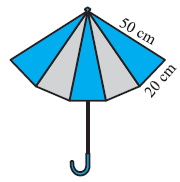Solution: Semi perimeter of each triangular piece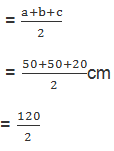=120/2 cm = 60cm

Using heron's formula,Area of the triangular piece

There are 5 colors in umbrella.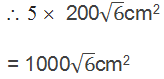Q7: A kite in the shape of a square with a diagonal 32 cm and an isosceles triangle of base 8 cm and sides 6 cm each is to be made of three different shades as shown in Fig. 12.17. How much paper of each shade has been used in it?

Solution: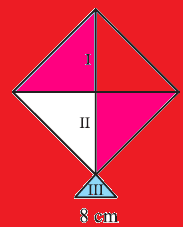As the diagonal of the square bisect each other at right angle.

Area of given kite = ½  (diagonal) 2

=   ½ × 32 × 32 = 512 cm2

Area of shade I = Area of shade II

512/2cm2 = 256 cm2

So, area of paper required in each shade = 256 cm2

For the III section,

Length of the sides of triangle = 6cm, 6cm and 8cm

Semi perimeter of triangle = (6 + 6 + 8)/2 cm = 10cm

Using heron's formula,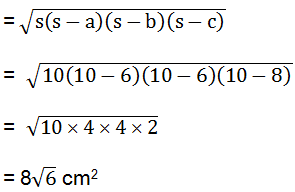Q8: A floral design on a floor is made up of 16 tiles which are triangular, the sides of the triangle being 9 cm, 28 cm and 35 cm (see Fig. 12.18).  Find the cost of polishing the tiles at the rate of 50p per cm2.

Solution:

Here a = 9cm

b = 28cm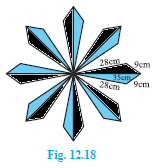c = 35cm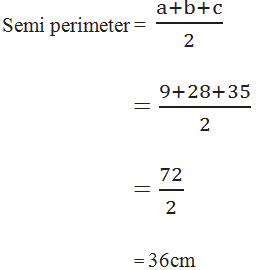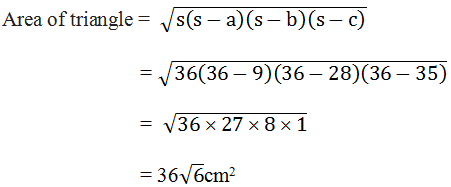= 36 ´ 2.45

= 88.2cm2

Area of 16 tiles = 16 ´ 88.2

= 1411.2cm2

Cost of polishing the tiles at the rate of 50p per cm2.

= 1411.2cm2 ´ 0.50

= Rs705.60

9. A field is in the shape of a trapezium whose parallel sides are 25 m and10 m. The non-parallel sides are 14 m and 13 m. find the area of the field.

Solution: Let ABCD be the given trapezium with parallel sides AB = 25m and CD = 10m and the non-parallel sides AD = 13m and BC = 14m.

CM ⊥ AB and CE || AD.

In ΔBCE,

BC = 14m, CE = AD = 13 m and

BE = AB - AE = 25 - 10 = 15m

Semi perimeter of the ΔBCE = (15 + 13 + 14)/2 m = 21 m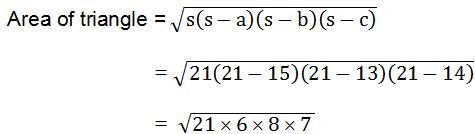= 84m2

Also, area of the ΔBCE = 1/2 × BE × CM = 84 m

⇒ 1/2 × 15 × CM = 84 m

⇒ CM = 168/15 m

⇒ CM = 56/5 m

Area of the parallelogram AECD = Base × Altitude

= AE × CM

= 10 × 84/5

= 112 m

Area of the trapezium ABCD = Area of AECD + Area of ΔBCE

= (112+ 84) m2

= 196 m

Page 2 of 2

Chapter Contents: